NEET  >  NCERT Exemplar: Electrochemistry

# NCERT Exemplar: Electrochemistry - Chemistry Class 12 - NEET

 Table of contentsMultiple Choice Questions - IMultiple Choice Questions - IIShort Answer Type QuestionsMatching TypeAssertion and Reason TypeLong Answer Type Questions## Multiple Choice Questions - I

Q.1. Which cell will measure standard electrode potential of copper electrode?
(i) Pt (s) | H2 (g,0.1 bar) | H+ (aq.,1 M) ║ Cu2+(aq.,1M) | Cu
(ii) Pt (s) | H2 (g, 1 bar) | H+ (aq.,1 M) ║ Cu2+ (aq.,2 M) | Cu
(iii) Pt (s) | H2 (g, 1 bar) | H+ (aq.,1 M) ║  Cu2+ (aq.,1 M) | Cu
(iv) Pt(s) | H2 (g, 1 bar) | H+ (aq.,0.1 M)  ║ Cu2+ (aq.,1 M) | Cu
Ans. (iii)
Solution.
Standard electrode potential of copper electrode can be calculated by constructing a concentration cell composed of two half cell reactions in which concentration of species on left hand and right hand side are unity. In such case cell potential is equal to standard, electrode potential.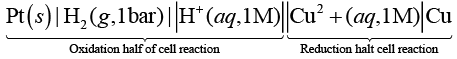Q.2. Electrode potential for Mg electrode varies according to the equation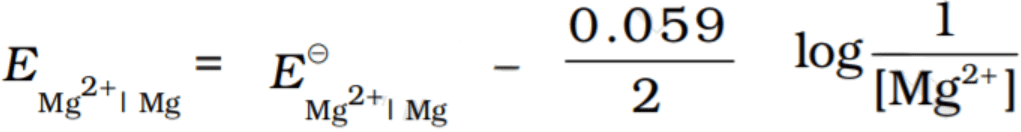. The graph of EMg+2| Mg  vs log [Mg2+] is
(i)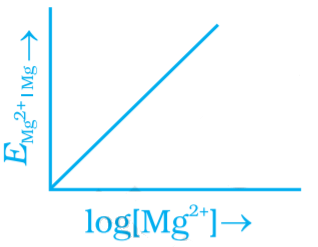(ii)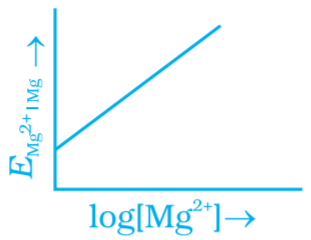(iii)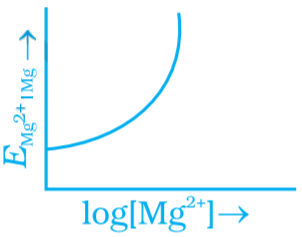(iv)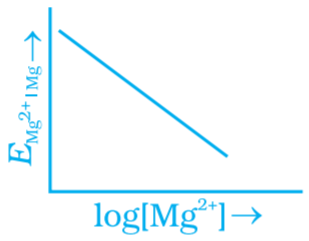Ans. (ii)
Sloution.
Compare this equation with the equation of straight line y = mx + c. The graph of EMg+2| Mg vs log[Mg+2] is a straight line with a positive slope and intercept EMg+2| Mg.

Q.3. Which of the following statement is correct?
(i) ECell and ∆rG of cell reaction both are extensive properties.
(ii) ECell and ∆rG of cell reaction both are intensive properties.
(iii) ECell is an intensive property while ∆rG of cell reaction is an extensive property.
(iv) ECell is an extensive property while ∆rG of cell reaction is an intensive property.
Ans. (iii)
Solution.
Ecell is an intensive property as it does not depend upon mass of species (number of particles) but ∆rG of the cell reaction is an extensive property because this depends upon mass of species (number of particles).

Q.4. The difference between the electrode potentials of two electrodes when no current is drawn through the cell is called ___________.
(i) Cell potential
(ii) Cell emf
(iii) Potential difference
(iv) Cell voltage
Ans. (ii)
Solution.
Cell emf: The difference between the electrode potential of two electrodes when no current is drawn through the cell is called cell emf.

Q.5. Which of the following statement is not correct about an inert electrode in a cell?
(i) It does not participate in the cell reaction.
(ii) It provides surface either for oxidation or for reduction reaction.
(iii) It provides surface for conduction of electrons.
(iv) It provides surface for redox reaction

Ans. (iv)
Solution.
An inert electrode in a cell provides surface for either oxidation or for reduction reaction by conduction of electrons through its surface but does not participate in the cell reaction. It does not provide surface for redox reaction.

Q.6. An electrochemical cell can behave like an electrolytic cell when ____________.
(i) Ecell = 0
(ii) Ecell > Eext
(iii) Eext > Ecell
(iv) Ecell = Eext
Ans. (iii)
Solution.
If an external opposite potential is applied on the galvanic cell and increased reaction continues to take place till the opposing voltage reaches the value 1.1V.
At this stage no current flow through the cell and if there is any further increase in the external potential then reaction starts functioning in opposite direction. Hence, this works as an electrolytic cell.

Q.7. Which of the statements about solutions of electrolytes is not correct?
(i) Conductivity of solution depends upon size of ions.
(ii) Conductivity depends upon viscosiy of solution.
(iii) Conductivity does not depend upon solvation of ions present in solution.
(iv) Conductivity of solution increases with temperature.
Ans. (iii)
Solution.
Conductivity does not depend upon solvation of ions present in solution Solution consists of electrolytes is known as electrolytic solution and conductivity of electrolytic solution depends upon the following factors
(i) Size of ions As ion size increases, ion mobility decreases and conductivity decreases.
(ii) Viscosity of solution Greater the viscosity of the solvent lesser will be the conductivity of the solution.
(iii) Solvation of ions Greater the solvation of ions of an electrolyte lesser will be the electrical conductivity of the solution.
(iv) Temperature of medium Conductivity of solution increases with increase in temperature.

Q.8. Using the data given below find out the strongest reducing agent.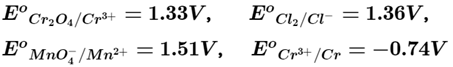(i) Cl
(ii) Cr
(iii) Cr3+
(iv) Mn2+
Ans. (ii)
Solution.
Here we use the concept of electrochemical series and standard reduction potential of the metal. Higher the negative value of standard reduction potential, strongest will be the reducing agent. Here, out of given four options standard reduction potential of chromium has highest negative value hence most powerful reducing agent is chromium.

Q.9. Use the data given in Q.8 and find out which of the following is the strongest oxidising agent.
(i) Cl
(ii) Mn2+
(iii) MnO4
(iv) Cr3+
Ans. (iii)
Solution.
Higher the positive value of standard reduction potential of metal ion higher will be its oxidising capacity. Since, E°MnO4-/Mn2+ has value equal to 1.51 V hence it is the strongest oxidising agent.

Q.10. Using the data given in Q.8 find out in which option the order of reducing power is correct.
(i) Cr3+ < Cl < Mn2+ < Cr
(ii) Mn2+ < Cl < Cr3+ < Cr
(iii) Cr3+ < Cl < Cr2O72– < MnO4
(iv) Mn2+ < Cr3+ < Cl < Cr
Ans. (ii)
Solution.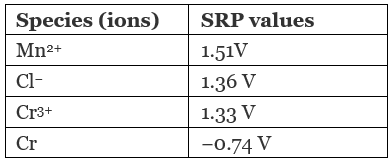On moving from top to bottom, standard reduction potential (SRP) value decreases from positive to negative which causes an increase in the reducing capacity of elements. So, the correct option is (ii).

Q.11. Use the data given in Q.8 and find out the most stable ion in its reduced form.
(i) Cl
(ii) Cr3+
(iii) Cr
(iv) Mn2+
Ans. (iv)
Solution.
MnO4-/Mn2+ has + ve value equal to 1.51 V which is highest among given four choices. So, Mn2+ is the most stable ion in its reduced form.

Q.12. Use the data of Q.8 and find out the most stable oxidised species.
(i) Cr3+
(ii) MnO4
(iii) Cr2O72–
(iv) Mn2+
Ans. (i)
Solution.
Cr3+ /Cr has most -ve value equal to -0.74 among given four choices. So, Cr3+ is the most stable oxidised species.

Q.13. The quantity of charge required to obtain one mole of aluminium from Al2O3 is ___________.
(i) 1F
(ii) 6F
(iii) 3F
(iv) 2F

Ans. (iii)
Solution.
The quantity of charge required to obtain one mole of aluminium from Al2O3 is equal to number of electrons required to convert Al2O3 to Al.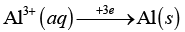Hence, total 3F charge is required.

Q.14. The cell constant of a conductivity cell _________.
(i) Changes with change of electrolyte.
(ii) Changes with change of concentration of electrolyte.
(iii) Changes with temperature of electrolyte.
(iv) Remains constant for a cell.
Ans. (iv)
Solution.
Remains constant for a cell
Cell constant is defined as the ratio of length of object and area of cross section.
G = l/A
Since, l and A remain constant for any particular object hence value of cell constant always remains constant.

Q.15. While charging the lead storage battery _________.
(i) PbSO4 anode is reduced to Pb.
(ii) PbSO4 cathode is reduced to Pb.
(iii) PbSO4 cathode is oxidised to Pb.
(iv) PbSO4 anode is oxidised to PbO2.
Ans. (i)
Solution.
While charging the lead storage battery the reaction occurring on cell is reversed and PbSO4 (s) on anode and cathode is converted into Pb and PbO2 respectively The electrode reactions are as follows:
At cathode PbSO4(s) + 2e → Pb(s) + SO42− (aq) (Reduction)
At anode PbSO4(s) + 2H2O → PbO2(s) + SO42− + 4H+ + 2e (Oxidation)
Overall reaction 2PbSO4(s) + 2H2O → Pb(s) + PbO2(s) + 4H+ (aq.) + 2SO42− (aq)

Q.16.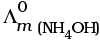is equal to ______________.
(i)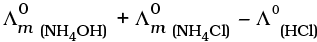(ii)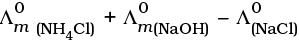(iii)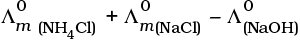(iv)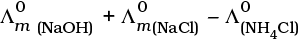Ans. (ii)
Solution.

According to Kohlrausch law limiting molar conductivity of any salt is equal to sum of limiting molar conductivity of individual molar conductivity of cations and anions of electrolyte.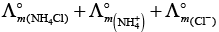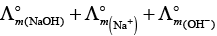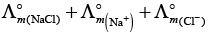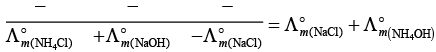Hence, option (ii) is correct choice.

Q.17. In the electrolysis of aqueous sodium chloride solution which of the half cell reaction will occur at anode?
(i) Na+ (aq) + e → Na (s); E°Cell = –2.71V
(ii) 2H2O (l) → O2 (g) + 4H+ (aq) + 4e ;  E°Cell = 1.23V
(iii) H+ (aq) + e → 1/2 H2 (g);  E°Cell = 0.00 V

(iv) Cl (aq) → 1/2 Cl2 (g) + e ;  E°Cell = 1.36 V
Ans. (iv)
Solution.

In case of electrolysis of aqueous NaCI oxidation reaction occurs at anode as follows
Cl (aq) → 1/2 Cl2(g) + e   E° = 1.36V
2H2O (I) → O2(g)+ 4H+(aq) + 4e   E°cell = 1.23 V
But due to lower E°cell value water should get oxidised in preference of Cl (aq).
However, the actual reaction taking place in the concentrated solution of NaCI is (iv) and not (ii) i.e., Cl2 is produced and not O2.
This unexpected result is explained on the basis of the concept of 'overvoltage', i.e., water needs greater voltage for oxidation to O2 (as it is kinetically slow process) than that needed for oxidation of Cr ions to CI2. Thus, the correct option is (iv) not (ii).

## Multiple Choice Questions - II

Note : In the following questions two or more than two options may be correct.
Q.18. The positive value of the standard electrode potential of Cu2+/Cu  indicates that ____________.
(i) This redox couple is a stronger reducing agent than the H+/H2 couple.
(ii) This redox couple is a stronger oxidising agent than H+/H2.
(iii) Cu can displace H2 from acid.
(iv) Cu cannot displace H2 from acid.
Ans. (ii,iv)
Solution.

'Lesser the E ° value of redox couple higher the reducing power"
Cu2+ + 2e → Cu    E° = 0.34V
2H + 2e → H2   E° = 0.00V
Since, 2H+/H2 has leaser SRP than Cu2+/Cu redox couple, Therefore,
(i) This redox couple is a stronger oxidising agent than H+/H2
(ii) Cu can't displace H2 from acid.
Hence, (ii) and (iv) are correct.

Q.19. E°Cell for some half cell reactions are given below. On the basis of these mark the correct answer.
(i) H+ (aq) + e → 1/2 H2 (g);  E°Cell = 0.00 V
(ii) 2H2O (l) → O2 (g) + 4H+ (aq) + 4e ;  E°Cell = 1.23V
(c) 2SO24 (aq) → S2O2–8 (aq) + 2e ; E°Cell = 1.96 V
(i) In dilute sulphuric acid solution, hydrogen will be reduced at cathode.
(ii) In concentrated sulphuric acid solution, water will be oxidised at anode.
(iii) In dilute sulphuric acid solution, water will be oxidised at anode.
(iv) In dilute sulphuric acid solution, SO42– ion will be oxidised to tetrathionate ion at anode.

Ans. (i, iii)
Solution.

During the electrolysis of dilute sulphuric acid above given three reaction occurs each of which represents particular reaction either oxidation half cell reaction or reduction half cell reaction.
Oxidation half cell reactions occur at anode are as follows
2SO42− (aq) → S2O82− + 2e−   cell = 1.96V
2H2O+ (l) → O2 (g) + 4H+ (aq) + 4e;    E°cell 1.23V
Reaction having lower value of E°cell will undergo faster oxidation.
Hence, oxidation of water occur preferentially reduction half ceil reaction occurs at cathode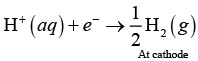Hence, options (i) and (ii) are correct.

Q.20. E°Cell = 1.1V  for Daniel cell. Which of the following expressions are correct description of state of equilibrium in this cell?
(i) 1.1 = Kc
(ii)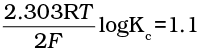(iii)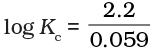(iv) log Kc = 1.1
Ans. (ii,iii)
Solution.

At state of equilibrium
ΔG = -RT log K
−nFE° = −RT2.303 log KC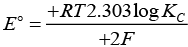[n = 2 for Daniel cell]
∵ At equilibrium E° =1.1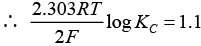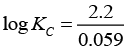[on solving]
Hence, options (ii) and (iii) are the correct choices.

Q.21. Conductivity of an electrolytic solution depends on ________.
(i) Nature of electrolyte.
(ii) Concentration of electrolyte.
(iii) Power of AC source.
(iv) Distance between the electrodes.
Ans. (i,ii)
Solution.

Conductivity of electrolytic solution is due to presence of mobile ions in the solution. This type of conductance is known as ionic conductance. Conductivity of these type of solutions depends upon
(i) the nature of electrolyte added
(ii) size of the ion produced and their solvatian
(iii) concentration of electrolyte (i) nature of solvent and its viscosity (ii) temperature
While power of source or distance between electrodes has no effect on conductivity of electrolyte solution. Hence, options (i) and (ii) are the correct choices.

Q.22.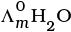is equal to _______________.
(i)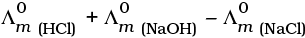(ii)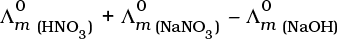(iii)(iv)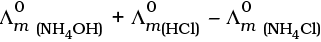Ans. (i,iii)
Solution.

This problem is based on the concept of Kohlrausch lawof independent migration of ions: is the sum of limiting molar conductivities of cation (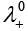) and anion (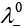) .
i.e.,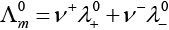(i)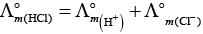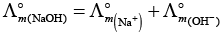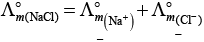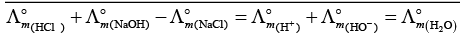(ii)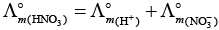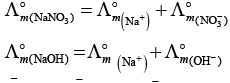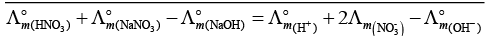(b) is incorrect
(iii)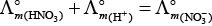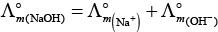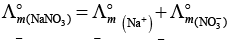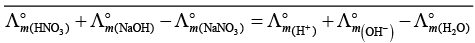Hence, Options (a) and (c) are the correct choices.
(iv)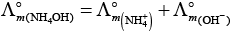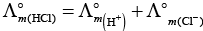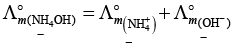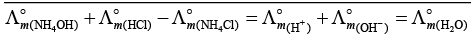This type of decomposition is not possible due to weak basic strength of NH4OH. This line will be placed above.

Q.23. What will happen during the electrolysis of aqueous solution of CuSO4 by using platinum electrodes?
(i) Copper will deposit at cathode.
(ii) Copper will deposit at anode.
(iii) Oxygen will be released at anode.
(iv) Copper will dissolve at anode.
Ans. (i,iii)
Solution.

For electrolysis of aqueous solution of CuSO4:
CuSO4 (aq) → CuH2++ SO42-
HO → 2H+ O2+
At anode 2O2- → O2 +2e-
At cathode Cu2+ + 2e → Cu(s)

Q.24. What will happen during the electrolysis of aqueous solution of  CuSO4 in the presence of Cu electrodes?
(i) Copper will deposit at cathode.
(ii) Copper will dissolve at anode.
(iii) Oxygen will be released at anode.
(iv) Copper will deposit at anode.
Ans. (i,ii)
Solution.

Electrolysis of CuSO4 can be represented by two half cell reactions these occurring at cathode and anode respectively as
At cathode Cu2++ 2e- → Cu(s)
At anode  Cu(s) → Cu2++ 2e
Here, Cu will deposit at cathode while copper will dissolved at anode.
Hence, options (a) and (b) are the correct choices.

Q.25. Conductivity κ , is equal to ____________.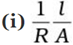(ii) G*/R
(iii) ∧m
(iv) l/A
Ans. (i,ii)
Solution.

As we know that, conductance is reciprocal of resistance and conductivity is reciprocal of resistivity.
k = 1/ρ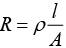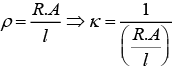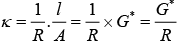Where, G* = Cell constant
Hence, options (i) and (ii) are the correct choices.

Q.26. Molar conductivity of ionic solution depends on ___________.
(i) Temperature.
(ii) Distance between electrodes.
(iii) Concentration of electrolytes in solution.
(iv) Surface area of electrodes.
Ans. (i,iii)
Solution.

Molar conductivity is the conductivity due to ions furnished by one mole of electrolyte in solution. Molar conductivity of ionic solution depends on
(i) Temperature: Molar conductivity of electrolyte solution increases with increase in temperature,
(ii) Concentration of electrolytes in solution: As concentration of electrolyte increases, molar conductivity decreases.

Q.27. For the given cell, Mg|Mg2+|| Cu2+|Cu
(i) Mg is cathode
(ii) Cu is cathode
(iii) The cell reaction is Mg + Cu2+→ Mg2+ + Cu
(iv) Cu is the oxidising agent
Ans. (ii,iii)
Solution.

Left side of cell reaction represents oxidation half cell i.e., oxidation of Mg and right side of cell represents reduction half cell reactions i.e., reduction of copper.

• Cu is reduced and reduction occurs at cathode.
• Mg is oxidised and oxidation occurs at anode

Whole cell reaction can be written as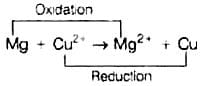Hence, options (ii) and (iii) both are correct choices.

Q.28. Can absolute electrode potential of an electrode be measured?
Ans. No, only the difference in potential between two electrodes can be measured. This is due to the reason that oxidation or reduction cannot occur alone. So, when we measure electrode potential of any electrode we have to take a reference electrode.

Q.29. Can E°Cell V or ∆rG° for cell reaction ever be equal to zero?
Ans. No, otherwise the reaction becomes non-feasible.
The reaction is feasible only at E°cell = positive or ∆rG° = negative.
When E° = ∆rG° = 0 the reaction reaches at equilibrium.

Q.30. Under what condition is E°Cell = 0 or ∆rG° = 0?
Ans. At the stage of chemical equilibrium in the cell we get E°cell = 0.
cell = 0
rG° = -n F E°cell
= −n x F x 0 = 0

Q.31. What does the negative sign in the expression E°Zn2+/Zn = − 0.76 V mean?
Ans. Greater the negative reactivity of standard reduction potential of metal greater is its reactivity. It means that Zn is more reactive than hydrogen. When zinc electrode will be connected to standard hydrogen electrode, Zn will get oxidised and H+ will get reduced.
Thus, zinc electrode will be the anode of the cell and hydrogen electrode will be the cathode of the cell.

Q.32. Aqueous copper sulphate solution and aqueous silver nitrate solution are electrolysed by 1 ampere current for 10 minutes in separate electrolytic cells. Will the mass of copper and silver deposited on the cathode be same or different? Explain your answer.
Ans. Different masses of Cu and Ag will be deposited at cathode. According to Faraday's second law of electrolysis amount of different substances liberated by same quantity of electricity passes through electrolyte solution is directly proportional to their chemical equivalent weight.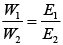Where, E1 and E2 have different values depending upon number of electrons required to reduce the metal ion. Thus, masses of Cu and Ag deposited will be different.

Q.33. Depict the galvanic cell in which the cell reaction is Cu + 2Ag+ → 2Ag + Cu2+
Ans. In a galvanic cell, oxidation half reaction is written on left hand side and reduction half reaction is on right hand side. Salt bridge is represented by parallel lines Cu | Cu2+ ||Ag+ | Ag.

Q.34. Value of standard electrode potential for the oxidation of Cl ions is more positive than that of water, even then in the electrolysis of aqueous sodium chloride, why is Cl oxidised at anode instead of water?
Ans. Under the condition of electrolysis of aqueous sodium chloride, oxidation of water at anode requires over potential. So, CI is oxidized at anode instead of water.
Possible oxidation half cell reactions occurring at anode are:
Cl(aq)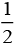Cl2 (g)+ e- ;   E°cell = 1.36V
2H2 O(l) O(g) 4H+ (aq) 4e- ; E°cell = 1.23V
Species having lower E°cell cell undergo oxidation first than the higher value but oxidation of H2O to O2 is kinetically so slow that it needs some overpotential.

Q.35. What is electrode potential?
Ans. The potential difference between the electrode and the electrolyte in an electrochemical cell is called electrode potential.

Q.36. Consider the following diagram in which an electrochemical cell is coupled to an electrolytic cell. What will be the polarity of electrodes ‘A’ and ‘B’ in the electrolytic cell?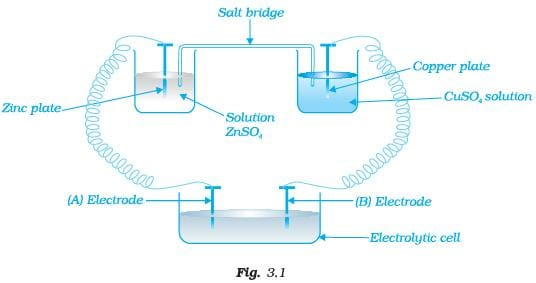Ans. The cell drawn above represents electrochemical cell in which two different electrodes are fitted in their respective electrolytic solutions and cell drawn at bottom represents electrolytic cell. Cell representation can be represented as Zn | Zn2+ || Cu2+|Cu.
Zn is losing electrons which are going towards electrode (A) and copper is accepting electron from electrode B. Hence,
Charge on electrode A = positive (+)
Charge on electrode B = negative (−)

Q.37. Why is alternating current used for measuring resistance of an electrolytic solution?
Ans. Alternating current is used in electrolysis so that concentration of ions in the solution remains constant and exact value of resistance is measured.

Q.38. A galvanic cell has electrical potential of 1.1V. If an opposing potential of 1.1V is applied to this cell, what will happen to the cell reaction and current flowing through the cell?

Ans. When an opposing potential of 1.1V is applied to a galvanic cell having electrical potential of 1.1V then cell reaction stops completely and no current will flow through the cell.

Q.39. How will the pH of brine (aq. NaCl solution) be affected when it is electrolysed?

Ans. The pH of the solution will rise as NaOH is formed in the electrolytic cell.
Chemical reactions occurring at cell when aqueous brine solution is electrolysed are as follows: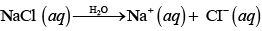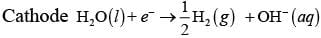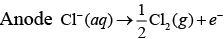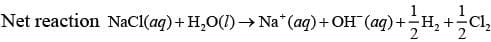Q.40. Unlike dry cell, the mercury cell has a constant cell potential throughout its useful life. Why?
Ans. Life time of any cell depends upon ions present in cell. Ions are not involved in the overall cell reaction of mercury cell. Hence, mercury cell has a constant cell potential throughout its useful life.

Q.41. Solutions of two electrolytes ‘A’ and ‘B’ are diluted. The Λm of ‘B’ increases 1.5 times while that of A increases 25 times. Which of the two is a strong electrolyte? Justify your answer.

Ans. Strong electrolytes dissociate almost completely even on high concentration. Therefore, concentration of such solutions remains almost same on dilution. Electrolyte 'B' is stronger than 'A' because in 'B' the number of ions remains the same on dilution, but only interionic attraction decreases.
Therefore, ∧m increases only 1.5 times, while in case of weak electrolyte on dilution, number of constituent ions increases.

Q.42. When acidulated water (dil.H2SO4 solution) is electrolysed, will the pH of the solution be affected? Justify your answer.
Ans. Since pH of solution depends upon concentration of H+present in solutions, pH of the solution will not be affected as [H+] remains constant.
At anode 2H2O → O2+ 4H+e-
At cathode 4H+ + 4e → 2H2

Q.43. In an aqueous solution how does specific conductivity of electrolytes change with addition of water?
Ans. Conductivity of solution due to total ions present in per unit volume of solution is known as specific conductivity. Specific conductivity decreases due to decrease in the number of ions per unit volume. We add water to aqueous solution, number of ions present in per unit volume decreases.

Q.44. Which reference electrode is used to measure the electrode potential of other electrodes?
Ans. Standard hydrogen electrode (SHE) is the reference electrode whose electrode potential is taken to be zero. The electrode potential of other electrodes are measured with respect to it.

Q.45. Consider a cell given below
Cu|Cu2+|| Cl-|Cl2,Pt
Write the reactions that occur at anode and cathode
Ans. Cell reaction represented in the question is composed of two half cell reactions. These reactions are as follows
At anode Cu → Cu2+ + 2e
At cathode Cl2 + 2e → 2Cl
Copper is getting oxidised at anode and Cl2 is getting reduced at cathode.

Q.46. Write the Nernst equation for the cell reaction in the Daniel cell. How will the ECell be affected when concentration of Zn2+ ions is increased?
Ans. Zn + Cu2 → Zn2+ Cu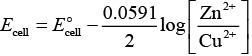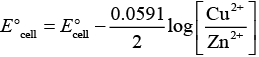According to this equation
cell is directly dependent on concentration of Cu2+ and inversely dependent upon concentration of Zn2+ ions.
Ecell decreases when concentration of Zn2+ ions is increased.

Q.47. What advantage do the fuel cells have over primary and secondary batteries?
Ans. Primary batteries contain a limited amount of reactants and are discharged when the reactants have been consumed. Secondary batteries can be recharged but it takes a long time. Fuel cell runs continuously as long as the reactants are supplied to it and products are removed continuously.

Q.48. Write the cell reaction of a lead storage battery when it is discharged. How does the density of the electrolyte change when the battery is discharged?
Ans. When a lead storage battery is discharged then the following cell reaction takes place
Pb + PbO2 + 2H2SO4 → 2PbSO4 + 2H2O
Density of electrolyte depends upon number of constituent ions present in per unit volume of electrolyte solution in this case density of electrolyte decreases as water is formed and sulphuric acid is consumed as the product during discharge of the battery.

Q.49. Why on dilution the Λm of CH3COOH increases drastically, while that of CH3COONa increases gradually?
Ans. In the case of CH3COOH, which is a weak electrolyte, the number of ions increases on dilution due to an increase in degree of dissociation.
CH3COOH + H2O ⇌ CH3COO+ H3O+
In case of strong electrolyte, the number of ions remains the same but the interionic attraction decreases.

## Matching Type

Note : Match the items of Column I and Column II in the following questions.
Q.50. Match the terms given in Column I with the units given in Column II.

 Column I Column II (i) ∧m (a) S cm–1 (ii) ECell (b) m-1 (iii) κ (c) S cm2 mol–1 (iv) G* = l/a (d) V

Ans. (i) → c, (ii) → d, (iii) → a, (iv)→ b
Solution.

 Column I Column II(Unit of parameter) (i) ∧m (c) S cm2 mol–1 (ii) ECell (d) V (iii) κ(conductivity) (a) S cm–1 (iv) G* = l/a (b) m-1

Q.51. Match the terms given in Column I with the items given in Column II.

 Column I Column II (i) Λm (a) Intensive property (ii) EºCell (b) Depends on number of ions/volume (iii) κ (c) Extensive property (iv) ∆rGCell (d) Increases with dilution

Ans. (i) → d, (ii) → a, (iii)→ b, (iv) → c
Solution.

(i) Λm (molar conductivity) is the conductivity due to number of ions furnished by one mole of electrolyte. As dilution increases number of ions present in the solution also increases hence molar conductivity increases.
(ii)Cell of any atom/ion does not depend upon number of atoms/ions. Hence EºCell of any atom/ion is an intensive properties.
(iii) κ represents specific conductivity which depends upon number of ions present in per unit volume.
(iv)rGCell is an extensive property as it depends upon number of particles (species)

Q.52. Match the items of Column I and Column II.

 Column I Column II (i) Lead storage battery (a) maximum efficiency (ii) Mercury cell (b) prevented by galvanisation (iii) Fuel cell (c) gives steady potential (iv) Rusting (d) Pb is anode, PbO2 is cathode

Ans. (i)→ d, (ii) → c, (iii)→ a, (iv)→ b
Solution.

(i) Chemical reaction occurring on lead storage battery can be represented as
At anode Pb(s) + SO42−(aq) → PbSO4 (s) + 2e
At cathode Pb(s) + SO42−(aq)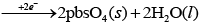Thus, Pb is anode and PbO2 is cathode.
(ii) Mercury cell does not include ions during their function hence produce steady current.
(iii) Fuel cell has maximum efficiency as they produce energy due to combustion reaction of fuel.
(iv) Rusting is prevented by corrosion.

Q.53. Match the items of Column I and Column II.

 Column I Column II (i) κ (a) I × t (ii) Λm (b) Λm/Λ0m (iii) α (c) κ/c (iv) Q (d) G*/R

Ans. (i) → d, (ii) → c, (iii)→ b, (iv) → a
Solution.

(i) Conductivity (k) =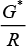(ii) Molar conductivity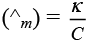(iii) Degree of dissociation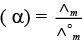(iv) Charge Q =I x t
where, Q is the quantity of charge in coulomb when I ampere of current is passed through an electrolyte for t second.

Q.54. Match the items of Column I and Column II.

 Column I Column II (i) Lechlanche cell (a) cell reaction 2H2 + O2 → 2H2O (ii) Ni–Cd cell (b) does not involve any ion in solution and is used in hearing aids. (iii) Fuel cell (c) rechargeable (iv) Mercury cell (d) reaction at anode, Zn → Zn2+ + 2e– (e) converts energy of combustion into electrical energy

Ans. (i)→ d, (ii) → c, (iii) → a, e (iv) → b
Solution.

(i) The electrode reaction occurring at Lechlanche cell are
At anode Zn(s) → Zn2 + 2e
At cathode MnO2NH4+ e → MnO (OH) NH3
(ii) Ni-Cd cell is rechargeable. So, it has more life time.
(iii) Fuel cell produces energy due to combustion. So, fuel cell converts energy of combustion into electrical energy e.g., 2H+ O2 → 2H2O
(iv) Mercury cell does not involve any ion in solution and is used in hearing aids.

Q.55. Match the items of Column I and Column II on the basis of data given below: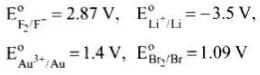Column I Column II (i) F2 (a) metal is the strongest reducing agent (ii) Li (b) metal ion which is the weakest oxidising agent (iii) Au3+ (c) non metal which is the best oxidising agent. (iv) Br– (d) unreactive metal (v) Au (e) anion that can be oxidised by Au3+ (vi) Li + (f) anion which is the weakest reducing agent (vii) F – (g) metal ion which is an oxidising agent

Ans. (i) → c, (ii)  → a, (iii) → g, (iv) → e, (v)  → d, (vi)  → b, (vii)  → f
Solution.

(i) F2 is a non-metal and best oxidising agent because SRP of F2 is + 2.87 V.
(ii) Li is a metal and strongest reducing agent because SRP of Li is −3.05 V.
(iii) Au3+ is a metal ion which is an oxidising agent as SRP of Au3+ is +1.40V.
(iv) Br is an anion that can be oxidised by
Au3+ as Au3+ (E° = 1.40) is greater than
Br (E° = 1,09V).
(v) Au is an unreactive metal.
(vi) Li+ is a metal ion having least value of SRP (− 3.05 V), hence it is the weakest oxidising agent.
(vii) F is an anion which is the weakest reducing agent as F /F2 has low oxidation potential (−2.87 V).

## Assertion and Reason Type

Note : In the following questions a statement of assertion followed by a statement of reason is given. Choose the correct answer out of the following choices.
(i) Both assertion and reason are true and the reason is the correct explanation of assertion.
(ii) Both assertion and reason are true and the reason is not the correct explanation of assertion.
(iii) Assertion is true but the reason is false.
(iv) Both assertion and reason are false.
(v) Assertion is false but reason is true.

Q.56. Assertion : Cu is less reactive than hydrogen.
Reason :Cu2+/Cu is negative.
(i) Both assertion and reason are true and the reason is the correct explanation of assertion.
(ii) Both assertion and reason are true and the reason is not the correct explanation of assertion.
(iii) Assertion is true but the reason is false.
(iv) Both assertion and reason are false.
(v) Assertion is false but reason is true.
Ans. (iii)
Solution.

Electrode potential of Cu2+ /Cu is + 0.34V and Electrode potential of 2H+ /H2 is 0.00 V.
Hence, correct reason is due to positive value of Cu2+ /Cu it looses electron to H+ and get reduces, while H2 gas evolves out.

Q.57. Assertion : ECell should have a positive value for the cell to function.
Reason : Ecathode < Eanode
(i) Both assertion and reason are true and the reason is the correct explanation of assertion.
(ii) Both assertion and reason are true and the reason is not the correct explanation of assertion.
(iii) Assertion is true but the reason is false.
(iv) Both assertion and reason are false.
(v) Assertion is false but reason is true.
Ans. (iii)
Solution.

Feasibility of chemical reaction depends on Gibbs free energy which is related to E°cell 3S
ΔG- = -nFE- cell
When value of E°cell is positive then ΔG° becomes negative. Hence, reaction becomes feasible, Correct reason is Ecathode > Eanode.

Q.58. Assertion : Conductivity of all electrolytes decreases on dilution.
Reason : On dilution number of ions per unit volume decreases.
(i) Both assertion and reason are true and the reason is the correct explanation of assertion.
(ii) Both assertion and reason are true and the reason is not the correct explanation of assertion.
(iii) Assertion is true but the reason is false.
(iv) Both assertion and reason are false.
(v) Assertion is false but reason is true.
Ans. (i)
Solution.

Since, conductivity depends upon number of ions per unit volume. Therefore, the conductivity of all electrolytes decreases on dilution due to decrease in number of ions per unit volume.

Q.59. Assertion : Λm for weak electrolytes shows a sharp increase when the electrolytic solution is diluted.
Reason : For weak electrolytes degree of dissociation increases with dilution of solution.
(i) Both assertion and reason are true and the reason is the correct explanation of assertion.
(ii) Both assertion and reason are true and the reason is not the correct explanation of assertion.
(iii) Assertion is true but the reason is false.
(iv) Both assertion and reason are false.
(v) Assertion is false but reason is true.
Ans. (i)
Solution.

Molar conductivity of weak electrolytic solution increases on dilution, because as we add excess water to increase the dilution degree of dissociation increases which leads to increase in number of ions in the solution. Thus, Λm show a very sharp increase.

Q.60. Assertion : Mercury cell does not give steady potential.
Reason : In the cell reaction, ions are not involved in solution.
(i) Both assertion and reason are true and the reason is the correct explanation of assertion.
(ii) Both assertion and reason are true and the reason is not the correct explanation of assertion.
(iii) Assertion is true but the reason is false.
(iv) Both assertion and reason are false.
(v) Assertion is false but reason is true.
Ans. (v)
Solution.

Assertion is false but reason is true, correct assertion is mercury cell gives steady potential.
Reason is correct as ions are not involved in cell reaction.

Q.61. Assertion : Electrolysis of NaCl solution gives chlorine at anode instead of O2.
Reason : Formation of oxygen at anode requires overvoltage.
(i) Both assertion and reason are true and the reason is the correct explanation of assertion.
(ii) Both assertion and reason are true and the reason is not the correct explanation of assertion.
(iii) Assertion is true but the reason is false.
(iv) Both assertion and reason are false.
(v) Assertion is false but reason is true.
Ans. (i)
Solution.

Electrolysis of NaCl is represented by following chemical reactions At cathode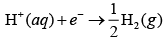At anode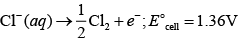2H2O(aq) O2(g) 4H+ (aq) 4e-; E°Cell 1.23V
E°cell for this reaction has lower value but formation of oxygen at anode requires over potential.

Q.62. Assertion : For measuring resistance of an ionic solution an AC source is used.
Reason : Concentration of ionic solution will change if DC source is used.
(i) Both assertion and reason are true and the reason is the correct explanation of assertion.
(ii) Both assertion and reason are true and the reason is not the correct explanation of assertion.
(iii) Assertion is true but the reason is false.
(iv) Both assertion and reason are false.
(v) Assertion is false but reason is true.
Ans. (i)
Solution.

Concentration of ionic solution changes on using DC current as a source of energy while on passing AC current concentration does not change. Hence, AC source is used for measuring resistance.

Q.63. Assertion : Current stops flowing when ECell = 0.
Reason : Equilibrium of the cell reaction is attained.
(i) Both assertion and reason are true and the reason is the correct explanation of assertion.
(ii) Both assertion and reason are true and the reason is not the correct explanation of assertion.
(iii) Assertion is true but the reason is false.
(iv) Both assertion and reason are false.
(v) Assertion is false but reason is true.
Ans. (i)
Solution.

Current stop flowing when Ecell = 0
As at Ecell = 0 reaction reaches the equilibrium.

Q.64. Assertion : EAg+/Ag increases with increase in concentration of Ag+ ions.
Reason : EAg+/Ag E has a positive value.
(i) Both assertion and reason are true and the reason is the correct explanation of assertion.
(ii) Both assertion and reason are true and the reason is not the correct explanation of assertion.
(iii) Assertion is true but the reason is false.
(iv) Both assertion and reason are false.
(v) Assertion is false but reason is true.
Ans. (ii)
Solution.

Both assertion and reason are correct but reason is not the correct explanation of assertion.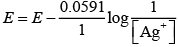E = E° + 0.059 log {Ag+]
Thus E Ag+/Ag increases with increase in concentration of Ag+

Q.65. Assertion : Copper sulphate can be stored in zinc vessel.
Reason : Zinc is less reactive than copper.
(i) Both assertion and reason are true and the reason is the correct explanation of assertion.
(ii) Both assertion and reason are true and the reason is not the correct explanation of assertion.
(iii) Assertion is true but the reason is false.
(iv) Both assertion and reason are false.
(v) Assertion is false but reason is true.
Ans. (iv)
Solution.

Copper sulphate can't be stored in zinc vessel as zinc is more reactive than copper due to negative value of standard reduction potential of Zn.

Q.66. Consider the Fig. 3.2 and answer the following questions.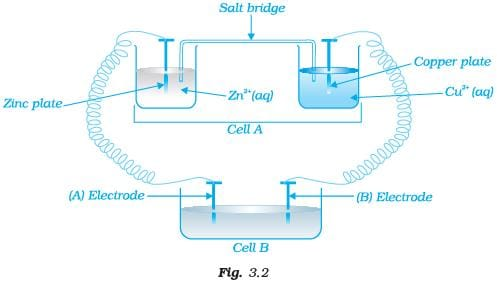(i) Cell ‘A’ has ECell = 2V and Cell ‘B’ has ECell = 1.1V which of the two cells ‘A’ or ‘B’ will act as an electrolytic cell. Which electrode reactions will occur in this cell?
(ii) If cell ‘A’ has ECell = 0.5V and cell ‘B’ has ECell = 1.1V then what will be the reactions at anode and cathode?

Ans. (i) Cell 'B' will act as electrolytic cell due to its lesser value of emf.
The electrode reactions will be
At cathode Zn2+ + 2e→ Zn
At anode Cu → Cu2+ + 2e
(ii) If cell 'B' has higher emf, it acts as galvanic cell.
Now it will push electrons into cell ‘A’
In this case, the reactions will be
Zn →Zn2+ + 2e (At anode)
Cu2+ +2e → Cu (At cathode)

Q.67. Consider Fig. 3.2 and answer the questions (i) to (vi) given below.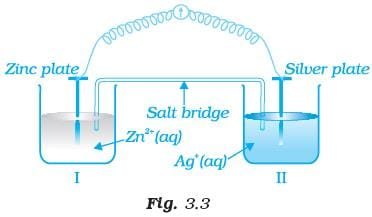(i) Redraw the diagram to show the direction of electron flow.
(ii) Is silver plate the anode or cathode?
(iii) What will happen if salt bridge is removed?
(iv) When will the cell stop functioning?
(v) How will concentration of Zn2+ ions and Ag+ ions be affected when the cell functions?
(vi) How will the concentration of Zn2+ ions and Ag+ ions be affected after the cell becomes ‘dead’?
Ans. (i) Electrons move from Zn to Ag as E' is more negative for Zn, so Zn undergoes oxidation and Ag+ undergoes reduction.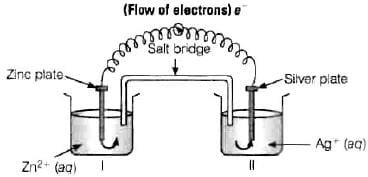(ii) Ag is the cathode as it is the site of reduction where Ag+ takes electrons from medium and deposit at cathode.
(iii) Cell will stop functioning because cell potential drops to zero. At E = 0 reaction reaches equilibrium.
(iv) When Ecell = 0 because at this condition reaction reaches to equilibrium.
(v) Concentration of Zn2+ ions will increase and concentration of Ag+ ions will decrease because Zn is converted into Zn2+ and Ag+ is converted into Ag.
(vi) When Ecell = 0 equilibrium is reached and concentration of Zn2+ ions and Agwill not change.

Q.68. What is the relationship between Gibbs free energy of the cell reaction in a galvanic cell and the emf of the cell? When will the maximum work be obtained from a galvanic cell?
Ans. If concentration of all reacting species is unity, then Ecell = E°cell and ΔG° = -nFE°cell where, ΔrG° is standard Gibbs energy of the reaction
cell = emf of the cell
nF = charge passed
If we want to obtain maximum work from a galvanic cell then charge has to be passed reversibly.
The reversibly work done by a galvanic cell is equal to decrease in its Gibbs energy.
ΔrG = -nFEcell
As Ecell is an intensive parameter but ΔrG is an extensive thermodynamic property and the value depends on n.
For reaction, Zn (s) + Cu2+ (aq) → Zn2+ (aq)+ Cu (s)in a galvanic cell.
ΔrG = -2FEcell [Here, n = 2]

The document NCERT Exemplar: Electrochemistry | Chemistry Class 12 - NEET is a part of the NEET Course Chemistry Class 12.
All you need of NEET at this link: NEET

## Chemistry Class 12

150 videos|377 docs|213 tests

## FAQs on NCERT Exemplar: Electrochemistry - Chemistry Class 12 - NEET

 1. What is electrochemistry?Ans. Electrochemistry is the branch of chemistry that deals with the study of the relationship between electricity and chemical reactions. It involves the use of electrochemical cells to convert chemical energy into electrical energy or vice versa.
 2. What are the applications of electrochemistry in everyday life?Ans. Electrochemistry has several applications in our daily lives. Some examples include: - Batteries: Electrochemical cells like batteries are used to power various portable devices such as smartphones, laptops, and electric vehicles. - Corrosion protection: Electroplating is used to provide a protective layer on metals, preventing them from corrosion. - Electrolysis: Electrolysis is used to extract metals from their ores, purify metals, and electroplate objects. - Fuel cells: Electrochemical cells called fuel cells are used to generate electricity by converting the energy released from the reaction between hydrogen and oxygen.
 3. What is a redox reaction in electrochemistry?Ans. A redox reaction, also known as a reduction-oxidation reaction, is a type of chemical reaction that involves the transfer of electrons between species. In electrochemistry, redox reactions are important as they are responsible for the generation of electricity in electrochemical cells. In a redox reaction, one species loses electrons (oxidation) while another species gains those electrons (reduction). The species that loses electrons is called the reducing agent, while the species that gains electrons is called the oxidizing agent.
 4. How does an electrochemical cell work?Ans. An electrochemical cell consists of two electrodes, an electrolyte, and a salt bridge. The two electrodes are placed in separate compartments, and the electrolyte allows the flow of ions between the electrodes. The salt bridge helps maintain electrical neutrality in the two compartments. When a redox reaction occurs in the electrochemical cell, electrons flow from the anode (where oxidation occurs) to the cathode (where reduction occurs) through an external circuit. This flow of electrons generates an electric current.
 5. What is the difference between a galvanic cell and an electrolytic cell?Ans. The main difference between a galvanic cell (also known as a voltaic cell) and an electrolytic cell is the source of electrical energy. In a galvanic cell, the redox reaction occurs spontaneously, and the chemical energy is converted into electrical energy. The anode is the site of oxidation, and the cathode is the site of reduction. In an electrolytic cell, electrical energy is used to drive a non-spontaneous redox reaction. The anode is the site of oxidation, and the cathode is the site of reduction. The purpose of an electrolytic cell is to induce a chemical change using electricity.

## Chemistry Class 12

150 videos|377 docs|213 testsExplore Courses for NEET exam### How to Prepare for NEET

Read our guide to prepare for NEET which is created by Toppers & the best Teachers
Signup to see your scores go up within 7 days! Learn & Practice with 1000+ FREE Notes, Videos & Tests.
10M+ students study on EduRev
Track your progress, build streaks, highlight & save important lessons and more!
Related Searches

,

,

,

,

,

,

,

,

,

,

,

,

,

,

,

,

,

,

,

,

,

;